# Interactive Geographical Maps with GeoPandas

Data VisualizationModelingGeoPandasposted by Parul Pandey December 23, 2021

The word geospatial is composed of two distinct terms. Geo means Earth, and Spatial means relating to or occupying space. Together it refers to the time-based...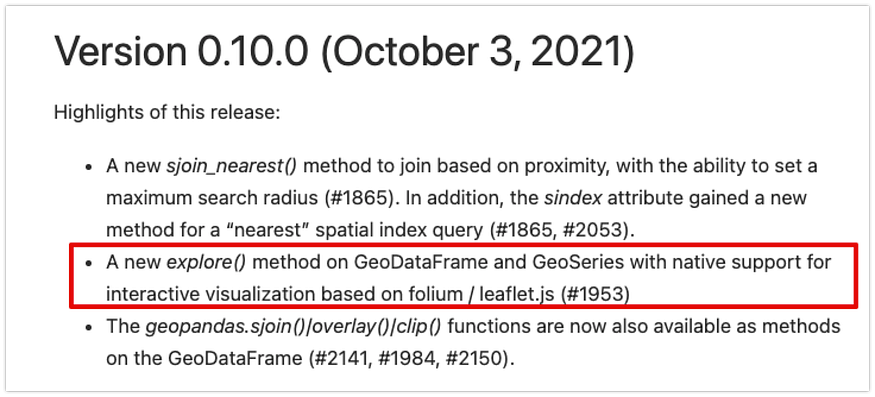Highlights of the latest release in GeoPandas library: Source: https://geopandas.readthedocs.io/en/latest/docs/changelog.html#version-0-10-0-october-3-2021

# Geopandas

## Installation

`conda install -c conda-forge geopandas`
`conda install -c conda-forge folium matplotlib mapclassifyorpip install folium matplotlib mapclassify`

## First steps with GeoPandas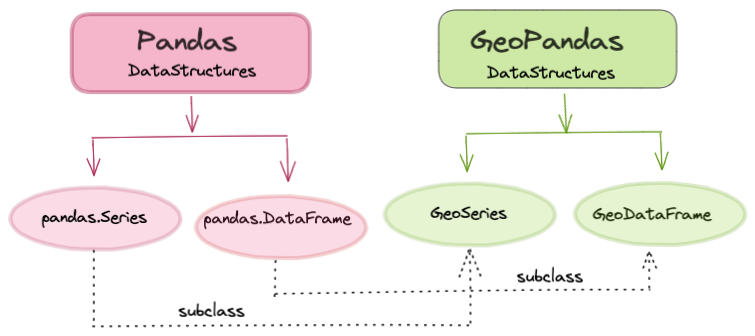GeoPandas builds up on Pandas library | Image by Author

## Importing the libraries

```import geopandas
import matplotlib.pyplot as plt
print(geopandas.__version__)
------------------------------------------
0.10.2```

```geopandas.datasets.available
----------------------------------------
['naturalearth_cities', 'naturalearth_lowres', 'nybb']```
```world_filepath = geopandas.datasets.get_path('naturalearth_lowres')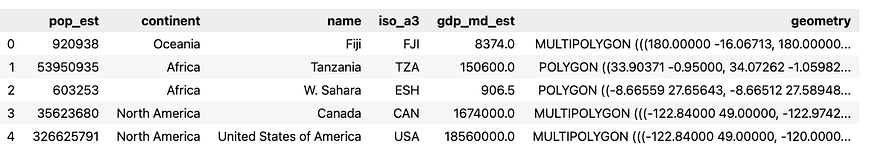A GeoPandas dataframe | Image by Author

## Visualizing the data

`world.plot()`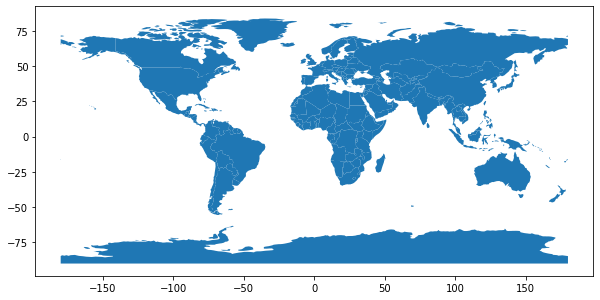Image by Author

```world.plot('pop_est', legend=True,figsize=(12,8))
plt.title('World Population')```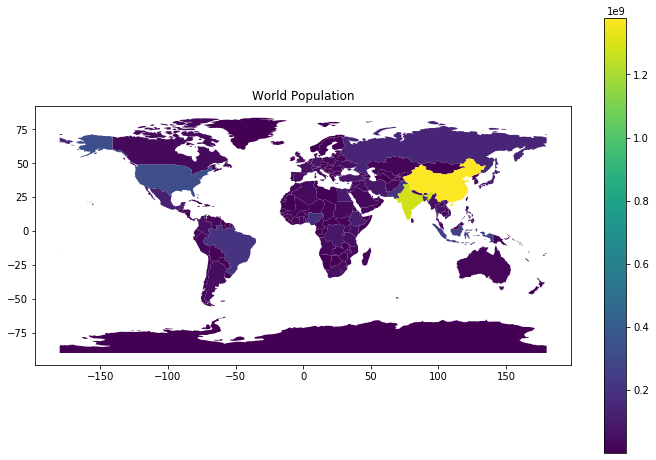Image by Author

```cmap='Set2'
world.plot('pop_est', cmap=cmap, legend=True,figsize=(12,8))
plt.title(f'World Population with colormap: {cmap}')

```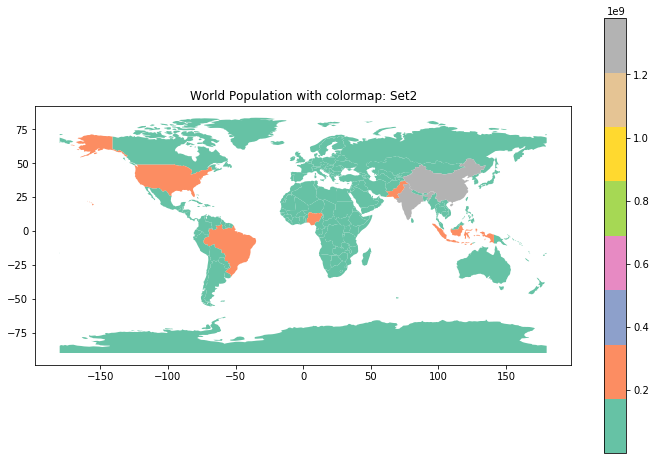Image by Author

```cmap='magma'
world.plot('pop_est', cmap=cmap, legend=True,figsize=(12,8))
plt.title(f'World Population with colormap: {cmap}')

```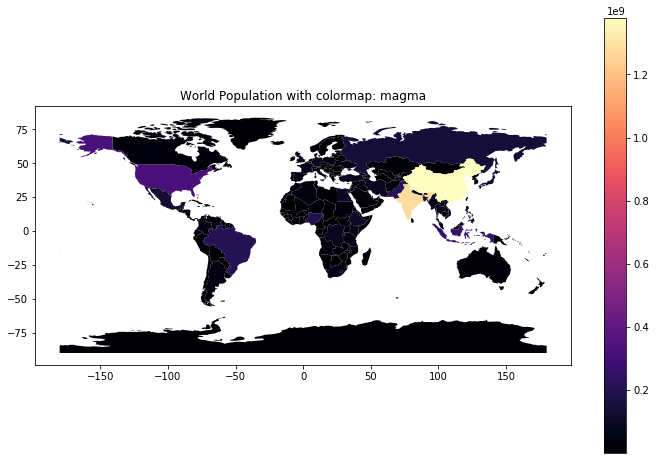Image by Author

• Boundaries
`world.boundary.plot(figsize=(12,8))`

# Plotting Interactive Maps

## Syntax

`GeoDataFrame.explore()`
`world.explore(column='pop_est',cmap='Set2')`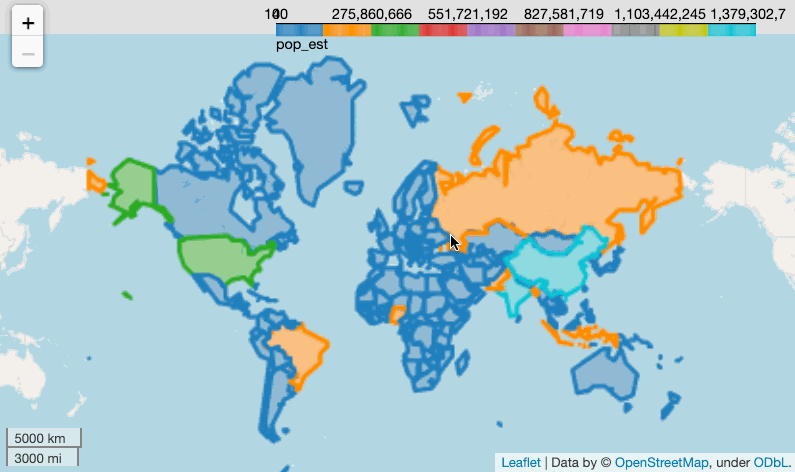Image by Author

```asia = world[world['continent'] =='Asia']
asia.explore(column='gdp_md_est',cmap='Set2')```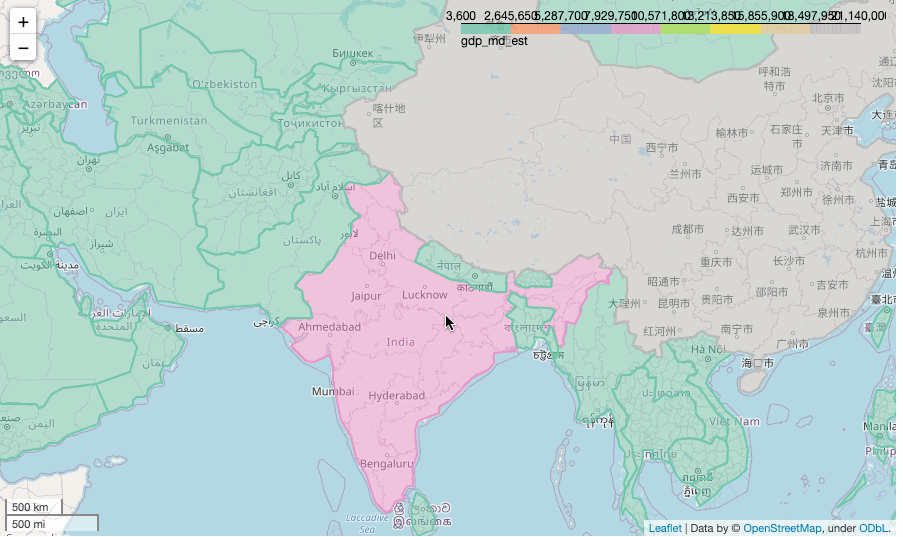Image by Author

## Parameters

```asia = world[world['continent'] =='Asia']
asia.explore(column='gdp_md_est',cmap='Set2',tooltip=False)```
```asia = world[world['continent'] =='Asia']
asia.explore(column='gdp_md_est',
cmap='Set2',
legend=False,
tooltip=['name','gdp_md_est'])```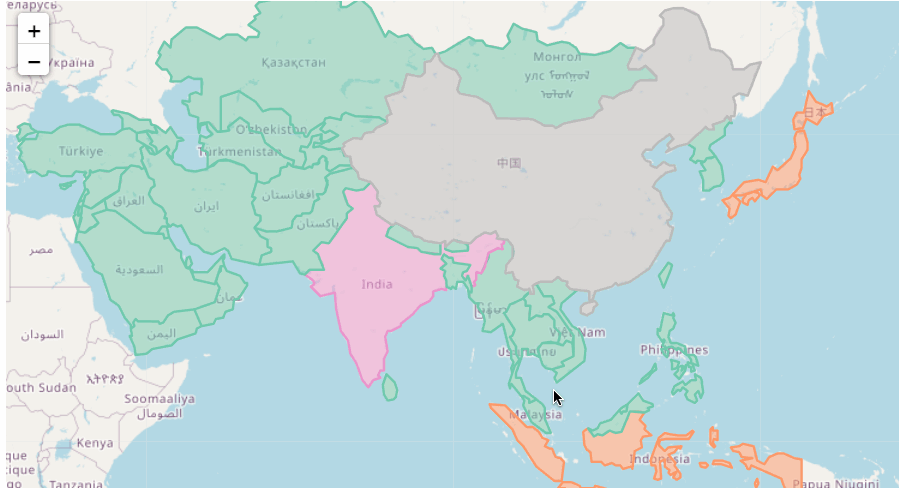Image by Author

```asia = world[world['continent'] =='Asia']
asia.explore(column='gdp_md_est',
cmap='Set2',
legend=False,
tooltip=False
popup=['name','gdp_md_est'])```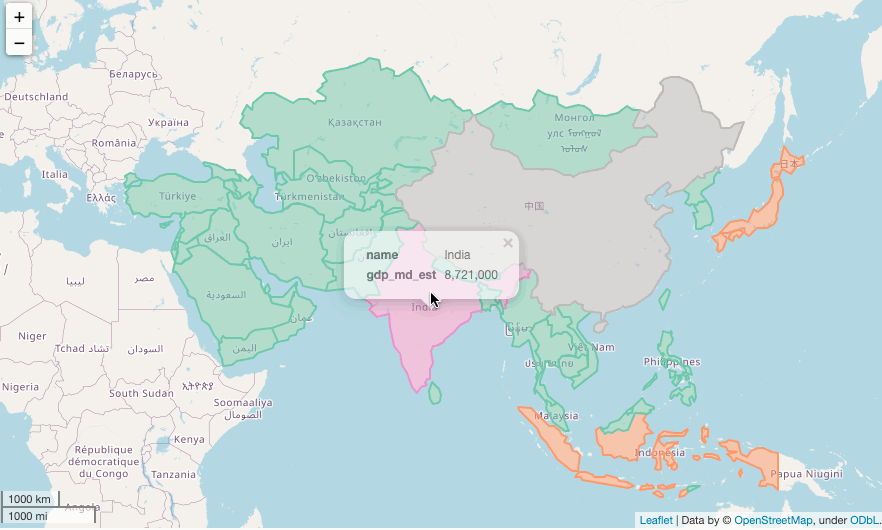Image by Author

```asia = world[world['continent'] =='Asia']asia.explore(column='gdp_md_est',
legend=False,
tiles=<enter the name of the tile to be used>)```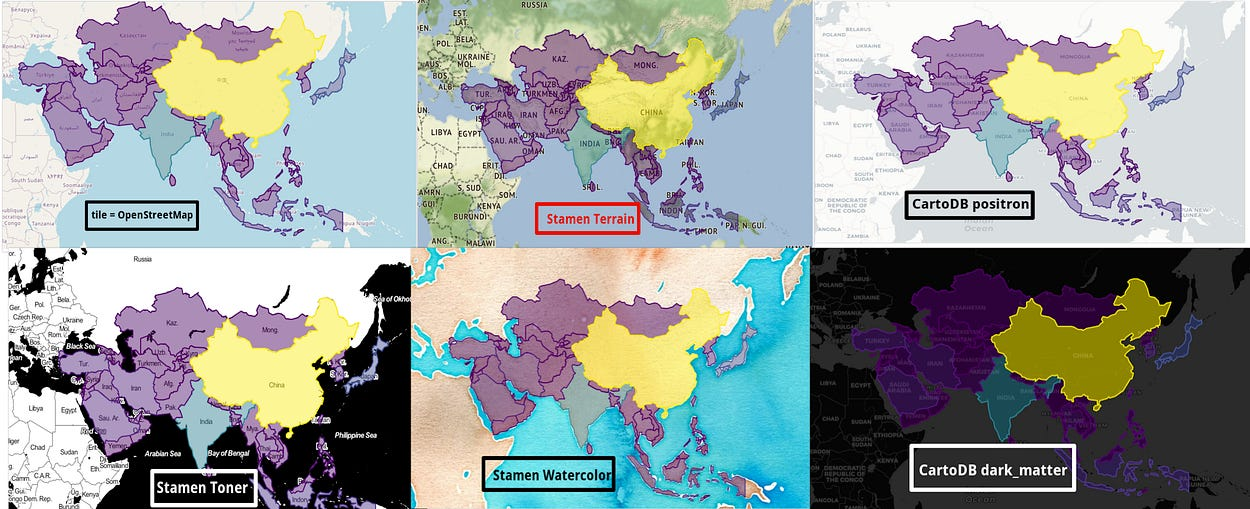Image by Author

```asia = world[world['continent'] =='Asia']
asia.explore(column='gdp_md_est',
cmap='Set2',
legend=False,
style_kwds=dict(color="black",weight=3, opacity=0.4))```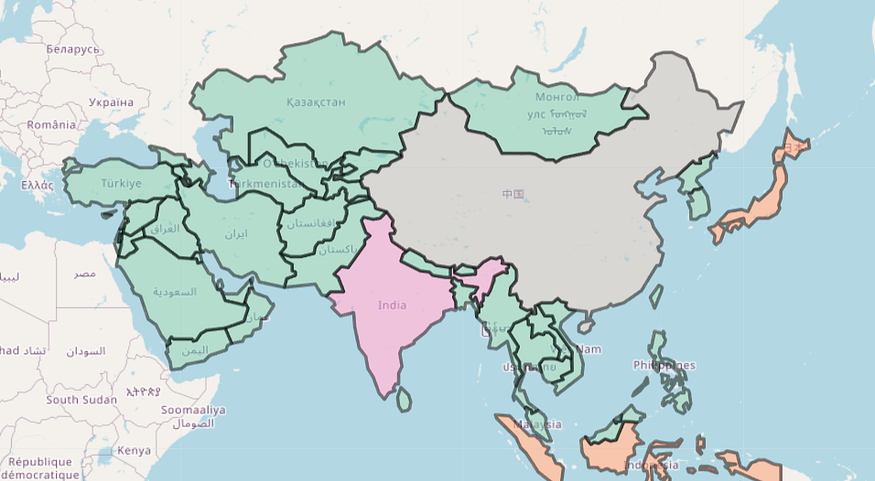Image by Author

## Visualizing India’s Seismic activity

This dataset includes a record of the date, time, location, depth, magnitude, and source of every Indian earthquake since 2018. Let’s import the data and look at the various attributes.```df = pd.read_csv('Indian_earthquake_data.csv')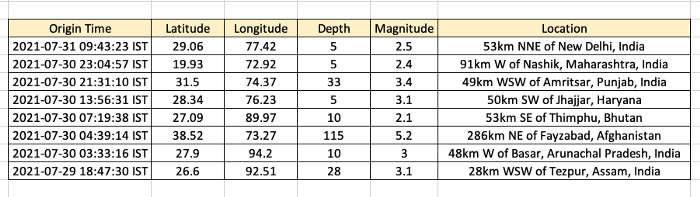```from geopandas import GeoDataFrame
from geopandas import points_from_xygeometry = points_from_xy(df['Latitude'],df['Longitude'])
df2 = GeoDataFrame(df, geometry=geometry)